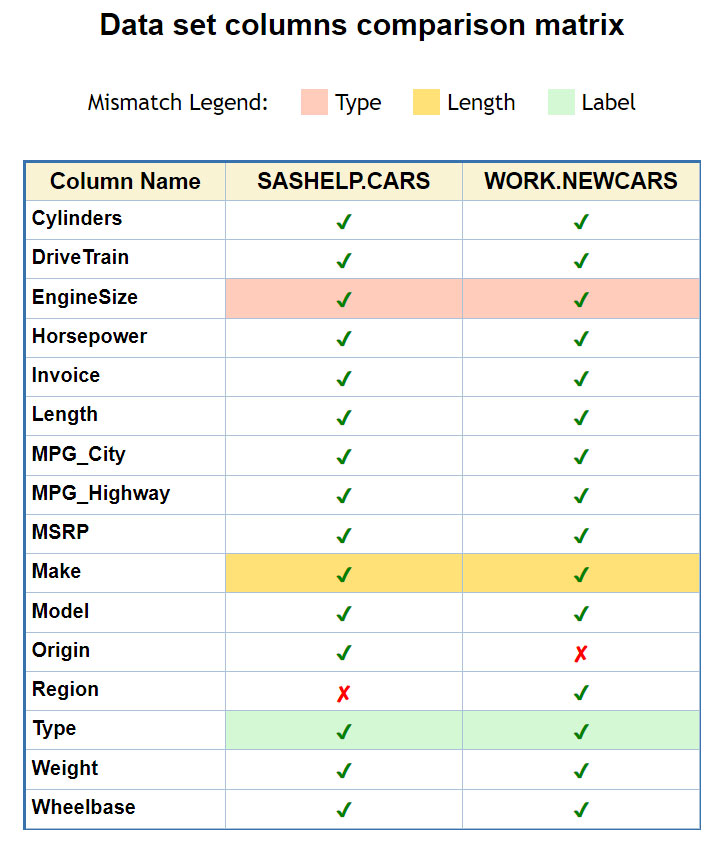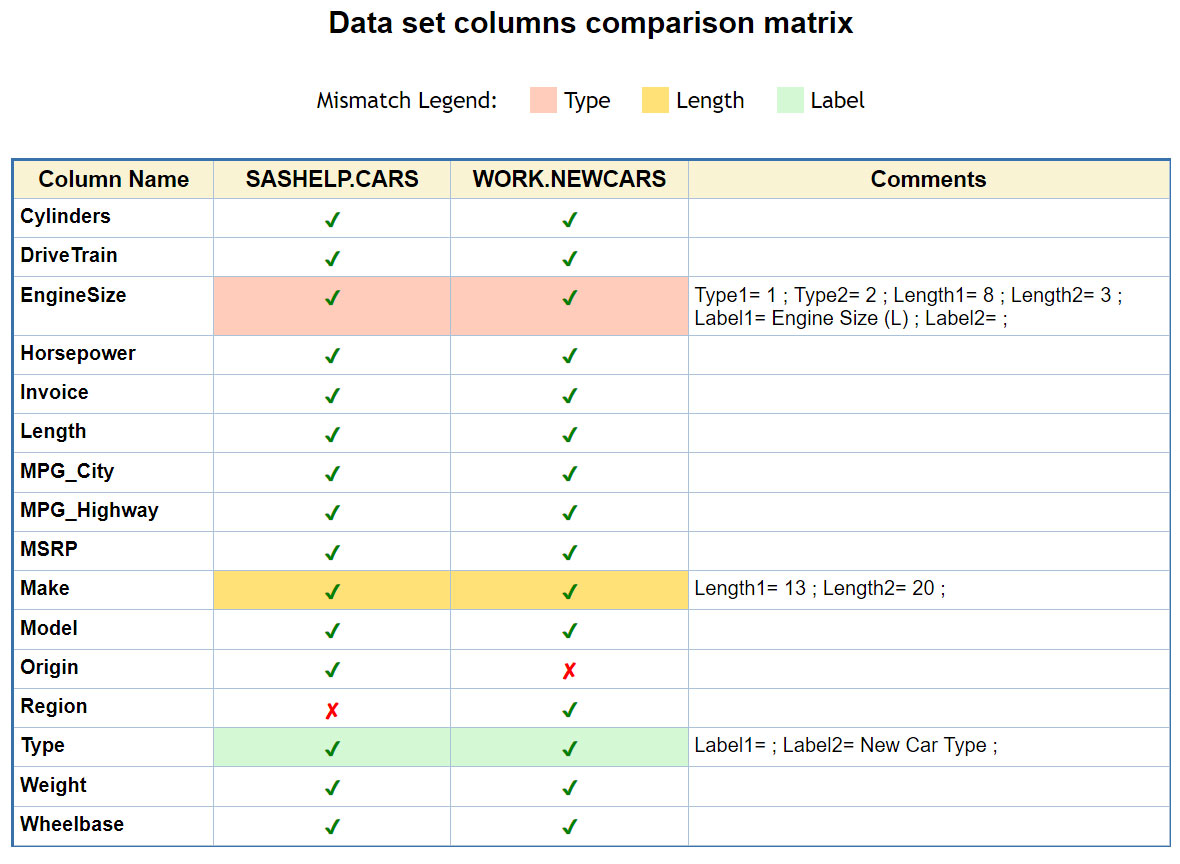Building on my last post, How to create checklist tables in SAS®, this one shows you how to compare SAS datasets that include common and uncommon columns. You'll learn how to visualize side-by-side columns commonalities and differences in data tables.

As before, we're working with a comparison matrix (aka checklist table) where check-marks / x-marks indicate included / excluded columns.

Data tables will be comparable products while their columns (variables) will represent product features. We'll add background color to highlight which attributes are different in the common columns. Since there might be several different attributes for a given column, we will use a hierarchy typelengthlabel to indicate only the highest mismatched level of hierarchy. For example:

• If same-named columns have different type (Numeric vs. Character), their corresponding check-mark will be shown on a light-red background, which indicates the highest degree of mismatch.
• If same-named columns have the same type, a yellow background will indicate any difference in variables length.
• When same-named variables type and length match, a light-blue background marks any difference in variables label.

## SAS code to create color-enhanced comparison matrix

Let’s compare variable attributes in two data tables: one is SAS-supplied SASHELP.CARS, and another WORK.NEWCARS that I derive from the first one, slightly scrambling its column definitions:

```data WORK.NEWCARS (drop=temp:); set SASHELP.CARS (rename=(Origin=Region EngineSize=temp1 Make=temp2)); length EngineSize \$3 Make \$20; EngineSize = put(temp1,3.1); Make = temp2; label Type='New Car Type'; run;```

In this NEWCARS data table, I did the following:

• Replaced column name Origin with Region
• Changed type of column EngineSize from Numeric to Character
• Changed length of column Make from \$13 to \$20
• Changed label of column Type from blank to “New Car Type”

Now let’s build the comparison matrix:

```proc contents data=SASHELP.CARS noprint out=DS1(keep=Name Type Length Label); run;   proc contents data=WORK.NEWCARS noprint out=DS2(keep=Name Type Length Label); run;   data comparison_matrix; merge DS1(in=in1 rename=(Type=Typ1 Length=Len1 Label=Lab1)) DS2(in=in2 rename=(Type=Typ2 Length=Len2 Label=Lab2)); by Name;   /* set symbol shape: 1=V; 0=X */ ds1 = 1; ds2 = 1; if in1 and not in2 then ds2 = 0; else if in2 and not in1 then ds1 = 0;   /* add background color */ if ds1=ds2=1 then select; when(Typ1^=Typ2) do; ds1=2; ds2=2; end; when(Len1^=Len2) do; ds1=3; ds2=3; end; when(Lab1^=Lab2) do; ds1=4; ds2=4; end; otherwise; end;   label Name = 'Column Name' ds1 = 'SASHELP.CARS' ds2 = 'WORK.NEWCARS' ; run;   proc format; value chmark 0 = '(*ESC*){unicode "2718"x}' 1-4 = '(*ESC*){unicode "2714"x}' ; value chcolor 0 = red 1-4 = green ; value bgcolor 2 = 'cxffccbb' 3 = 'cxffe177' 4 = 'cxd4f8d4' ; run;   ods html path='c:\temp' file='comp_marix.html' style=Seaside; ods escapechar='^'; title 'Data set columns comparison matrix';   proc odstext; p '<div align="center">Mismatch Legend:'|| '<span style="background-color:#ffccbb;margin-left:17px">^_^_^_^_</span> Type'|| '<span style="background-color:#ffe177;margin-left:17px">^_^_^_^_</span> Length'|| '<span style="background-color:#d4f8d4;margin-left:17px">^_^_^_^_</span> Label</div>' / style=[fontsize=9pt]; run;   title; proc print data=comparison_matrix label noobs; var Name / style={fontweight=bold width=100px}; var ds1 ds2 / style={color=chcolor. backgroundcolor=bgcolor. just=center fontweight=bold width=120px}; format ds1 ds2 chmark.; run;   ods html close;```

Here is a brief explanation of the code:

1. Two PROC CONTENTS produce alphabetical lists (as datasets) of the data table column names, as well as their attributes (type, length, label)
2. The DATA STEP merges these 2 lists and creates DS1 and DS2 variables indicating common name (values 1, 2, 3, 4) or uncommon name (value 0).
3. PROC FORMAT creates 3 user-defined formats chmark, chcolor, bgcolor responsible for checkmark shape, checkmark color, and background color respectively. For checkmark shape, we use Unicode characters, and for colors we use both, color names (e.g. red, green) and hexadecimal RGB color notations (e.g. 'cxFFCCBB').
4. PROC ODSTEXT’s P statement is used to display color legend for the comparison matrix.
5. Finally, PROC PRINT with user-defined formats produces our color-enhanced comparison matrix.

## Data tables comparison matrix – OUTPUT

The above code will generate the following HTML output with the comparison matrix for variables in two data sets:## Adding more detail to the comparison matrix chart

We can further enhance our output comparison matrix by adding detailed descriptive information about differences between variable attributes. For comprehensive view, we can add a COMMENTS column that spells out differences (attributes mismatches). In addition to the hierarchical logic defining only one mismatch of the highest degree indicated by color highlighting above, comments can include all found discrepancies. Simply add the following two pieces of SAS code:

1. Add the following group of statements to the above DATA Step (right after SELECT statement):

``` length Comments \$200; if ds1>1 then do; if Typ1^=Typ2 then Comments = catx(' ', Comments, 'Type1=', Typ1, '; Type2=', Typ2, ';'); if Len1^=Len2 then Comments = catx(' ', Comments, 'Length1=', Len1, '; Length2=', Len2, ';'); if Lab1^=Lab2 then Comments = catx(' ', Comments, 'Label1=', Lab1, '; Label2=', Lab2, ';'); end;```

Depending on your needs this Comments can be added unconditionally – you would just need to remove IF-THEN logic keeping only:

```length Comments \$200; Comments = catx(' ', Comments, 'Type1=', Typ1, '; Type2=', Typ2, ';'); Comments = catx(' ', Comments, 'Length1=', Len1, '; Length2=', Len2, ';'); Comments = catx(' ', Comments, 'Label1=', Lab1, '; Label2=', Lab2, ';');```

2. Add the following statement to the above PROC PRINT (right before the FORMAT statement):

Then your HTML output will look as follows:## Conclusion

Comparison matrix charts are a convenient tool for data development and metadata validation when you're comparing a data table’s metadata against requirements descriptions.

It allows us to quickly identify tables’ common and uncommon variables, as well as common variable inconsistencies by type, length and other attributes, such as labels and formats.

We can easily add detailed descriptive information when needed.

## On a related note

While this post focused on visualizing SAS data sets comparison vis-à-vis common and uncommon columns, it's worth noting SAS websites have plenty of info on finding common variables (or columns) in data sets. For example: